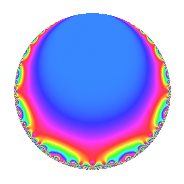# Properties

 Label 600.2.a.cLevel 600 Weight 2 Character orbit 600.a Self dual Yes Analytic conductor 4.791 Analytic rank 1 Dimension 1 CM No Inner twists 1

# Related objects

## Newspace parameters

 Level: $$N$$ = $$600 = 2^{3} \cdot 3 \cdot 5^{2}$$ Weight: $$k$$ = $$2$$ Character orbit: $$[\chi]$$ = 600.a (trivial)

## Newform invariants

 Self dual: Yes Analytic conductor: $$4.79102412128$$ Analytic rank: $$1$$ Dimension: $$1$$ Coefficient field: $$\mathbb{Q}$$ Coefficient ring: $$\mathbb{Z}$$ Coefficient ring index: $$1$$ Fricke sign: $$1$$ Sato-Tate group: $\mathrm{SU}(2)$

## $q$-expansion

 $$f(q)$$ $$=$$ $$q$$ $$\mathstrut -\mathstrut q^{3}$$ $$\mathstrut +\mathstrut q^{9}$$ $$\mathstrut +\mathstrut O(q^{10})$$ $$q$$ $$\mathstrut -\mathstrut q^{3}$$ $$\mathstrut +\mathstrut q^{9}$$ $$\mathstrut -\mathstrut 4q^{11}$$ $$\mathstrut -\mathstrut 6q^{13}$$ $$\mathstrut +\mathstrut 6q^{17}$$ $$\mathstrut -\mathstrut 4q^{19}$$ $$\mathstrut -\mathstrut q^{27}$$ $$\mathstrut -\mathstrut 2q^{29}$$ $$\mathstrut -\mathstrut 8q^{31}$$ $$\mathstrut +\mathstrut 4q^{33}$$ $$\mathstrut +\mathstrut 2q^{37}$$ $$\mathstrut +\mathstrut 6q^{39}$$ $$\mathstrut -\mathstrut 6q^{41}$$ $$\mathstrut -\mathstrut 12q^{43}$$ $$\mathstrut -\mathstrut 8q^{47}$$ $$\mathstrut -\mathstrut 7q^{49}$$ $$\mathstrut -\mathstrut 6q^{51}$$ $$\mathstrut -\mathstrut 6q^{53}$$ $$\mathstrut +\mathstrut 4q^{57}$$ $$\mathstrut +\mathstrut 12q^{59}$$ $$\mathstrut +\mathstrut 14q^{61}$$ $$\mathstrut -\mathstrut 4q^{67}$$ $$\mathstrut +\mathstrut 8q^{71}$$ $$\mathstrut +\mathstrut 6q^{73}$$ $$\mathstrut -\mathstrut 8q^{79}$$ $$\mathstrut +\mathstrut q^{81}$$ $$\mathstrut +\mathstrut 12q^{83}$$ $$\mathstrut +\mathstrut 2q^{87}$$ $$\mathstrut +\mathstrut 10q^{89}$$ $$\mathstrut +\mathstrut 8q^{93}$$ $$\mathstrut -\mathstrut 2q^{97}$$ $$\mathstrut -\mathstrut 4q^{99}$$ $$\mathstrut +\mathstrut O(q^{100})$$

## Embeddings

For each embedding $$\iota_m$$ of the coefficient field, the values $$\iota_m(a_n)$$ are shown below.

For more information on an embedded modular form you can click on its label.

Label $$\iota_m(\nu)$$ $$a_{2}$$ $$a_{3}$$ $$a_{4}$$ $$a_{5}$$ $$a_{6}$$ $$a_{7}$$ $$a_{8}$$ $$a_{9}$$ $$a_{10}$$
1.1
 0
0 −1.00000 0 0 0 0 0 1.00000 0
 $$n$$: e.g. 2-40 or 990-1000 Significant digits: Format: Complex embeddings Normalized embeddings Satake parameters Satake angles

## Inner twists

This newform does not admit any (nontrivial) inner twists.

## Atkin-Lehner signs

$$p$$ Sign
$$2$$ $$1$$
$$3$$ $$1$$
$$5$$ $$1$$

## Hecke kernels

This newform can be constructed as the intersection of the kernels of the following linear operators acting on $$S_{2}^{\mathrm{new}}(\Gamma_0(600))$$:

 $$T_{7}$$ $$T_{11}$$ $$\mathstrut +\mathstrut 4$$